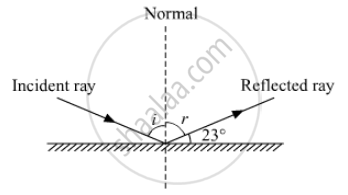SSC (English Medium) Class 8Maharashtra State Board
Share

# Solve the Following Example - SSC (English Medium) Class 8 - Science

ConceptConcept of Reflection of Light

#### Question

Solve the following example.

If the angle between the mirror and reflected ray is 23, what is the angle of incidence of the incidence ray?

#### Solutionangle r + 23° = 90°

=> angle r = 67°

Using law of reflection , we have

angle i = angle r = 67°

Is there an error in this question or solution?

#### APPEARS IN

Balbharati Solution for Balbharati Class 8 Science - General Science (2018 to Current)
Chapter 16: Reflection of Light
Exercise | Q: 7.2 | Page no. 115
Solution Solve the Following Example Concept: Concept of Reflection of Light.
S